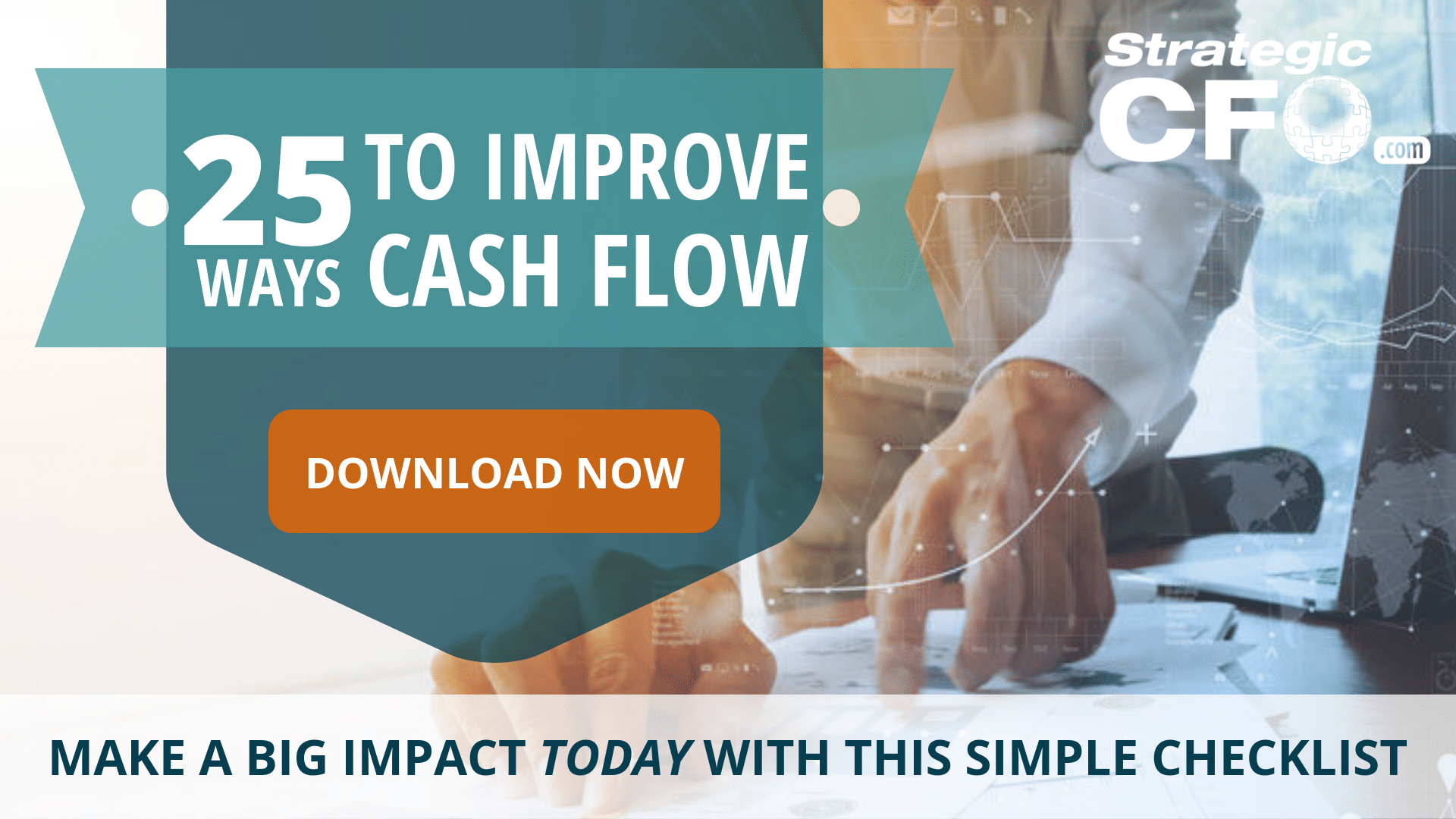# Cash Flow After Tax

In finance, analysts calculate after-tax cash flows to determine the cash flows of an investment or corporate project.

Basically, the analyst calculates the after tax earnings of the investment or project, and then adds back the depreciation charge. Depreciation s counted as a cost that acts as a shield to diminish the tax effect. Then the depreciation charge is added back to after-tax earnings because it is a non-cash expense. Depreciation represents the declining economic value of an asset, but is not an actual cash outflow.

## Cash Flow After Tax Formula

Use the following cash flow after tax formula:

After Tax Cash Flow = Earnings After Tax + Depreciation

(NOTE: Want the 25 Ways To Improve Cash Flow? It gives you tips that you can take to manage and improve your company’s cash flow in 24 hours!. Get it here!)

### Cash Flow After Tax Example

Let’s say a financial analyst must calculate the cash flow after tax of a corporate project with operating income of \$20 million dollars, a depreciation charge of \$3 million dollars, and a tax rate of 40%.

First, the financial analyst would subtract the depreciation charge of \$3 million dollars from the operating income of \$20 million dollars. This would result in a profit before tax of \$17 million dollars.

Then the financial analyst would calculate the effects of the tax charge by multiplying \$17 million dollars by 60%, or one minus the tax rate, resulting in an after-tax income of \$10.2 million dollars.

And finally, the analyst adds back the depreciation charge of \$3 million dollars. While the depreciation charge has a real effect on taxes, and is thus a relevant figure, it is not an actual cash outflow, so it must be added back to after-tax income. So the cash flow after tax is \$13.2 million dollars.

```Operating Income            \$20
Less Depreciation             3
Profit Before Tax            17
Less Tax Charge             6.8
Income After Tax           10.2
Plus Depreciation             3
Cash Flow After Tax       \$13.2
```

For more ways to improve your cash flow, download the free 25 Ways to Improve Cash Flow whitepaper.Strategic CFO Lab Member Extra

Access your Cash Flow Tuneup Execution Plan in SCFO Lab. This tool enables you to quantify the cash unlocked in your company.